# JP2006041878A - Signal processor and signal processing method for discrete signal - Google Patents

## Info

Publication number
JP2006041878A
JP2006041878A JP2004218460A JP2004218460A JP2006041878A JP 2006041878 A JP2006041878 A JP 2006041878A JP 2004218460 A JP2004218460 A JP 2004218460A JP 2004218460 A JP2004218460 A JP 2004218460A JP 2006041878 A JP2006041878 A JP 2006041878A
Authority
JP
Japan
Prior art keywords
signal
value
sampling
function
sample
Prior art date
Legal status (The legal status is an assumption and is not a legal conclusion. Google has not performed a legal analysis and makes no representation as to the accuracy of the status listed.)
Granted
Application number
JP2004218460A
Other languages
Japanese (ja)
Other versions
JP3808483B2 (en
Inventor
Kazuoki Katagishi
Toru Kawabe
Koji Nakamura
Tomoyuki Takahashi
Kazuo Toraichi

Original Assignee
Japan Science & Technology Agency

Priority date (The priority date is an assumption and is not a legal conclusion. Google has not performed a legal analysis and makes no representation as to the accuracy of the date listed.)
Filing date
Publication date
Application filed by Japan Science & Technology Agency, 独立行政法人科学技術振興機構 filed Critical Japan Science & Technology Agency
Priority to JP2004218460A priority Critical patent/JP3808483B2/en
Publication of JP2006041878A publication Critical patent/JP2006041878A/en
Application granted granted Critical
Publication of JP3808483B2 publication Critical patent/JP3808483B2/en
Application status is Active legal-status Critical
Anticipated expiration legal-status Critical

## Images

••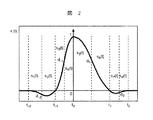•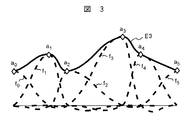•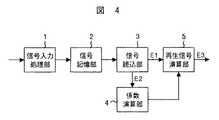•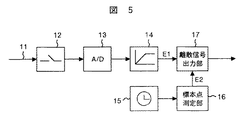•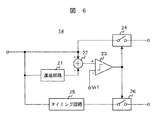•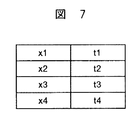•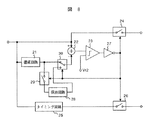•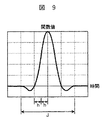## Classifications

• HELECTRICITY
• H03BASIC ELECTRONIC CIRCUITRY
• H03MCODING; DECODING; CODE CONVERSION IN GENERAL
• H03M1/00Analogue/digital conversion; Digital/analogue conversion
• H03M1/66Digital/analogue converters
• H03M1/661Improving the reconstruction of the analogue output signal beyond the resolution of the digital input signal, e.g. by interpolation, by curve-fitting, by smoothing
• HELECTRICITY
• H03BASIC ELECTRONIC CIRCUITRY
• H03MCODING; DECODING; CODE CONVERSION IN GENERAL
• H03M1/00Analogue/digital conversion; Digital/analogue conversion
• H03M1/12Analogue/digital converters
• H03M1/124Sampling or signal conditioning arrangements specially adapted for A/D converters
• H03M1/1245Details of sampling arrangements or methods
• H03M1/1265Non-uniform sampling
• HELECTRICITY
• H03BASIC ELECTRONIC CIRCUITRY
• H03MCODING; DECODING; CODE CONVERSION IN GENERAL
• H03M7/00Conversion of a code where information is represented by a given sequence or number of digits to a code where the same, similar or subset of information is represented by a different sequence or number of digits
• H03M7/30Compression; Expansion; Suppression of unnecessary data, e.g. redundancy reduction

## Abstract

To provide a signal processing apparatus and a signal processing method capable of performing smooth signal reproduction in reproducing a discrete signal with nonuniform sampling point intervals.
A coefficient calculation unit 4 that receives a sampling point signal E2 representing the time of sampling points of a discrete signal E1 with nonuniform sampling point intervals and obtains a coefficient of a sampling function corresponding to the discrete signal; a discrete signal; And a reproduction signal calculation unit 5 that obtains a continuous reproduction signal E3 by calculating and outputting a function value within the sampling point interval from the coefficient value output by the coefficient calculation unit.
[Selection] Figure 1

## Description

The present invention relates to a processing apparatus and a processing method effective for discretization of a continuous signal and reproduction of the discretized signal, and is particularly suitable for application to an image signal, an acoustic signal, or a signal obtained by extracting an outline of an image. The present invention relates to a signal processing device and a signal processing method.

The discretization of the continuous signal is performed by a sampler (sampling circuit) that extracts the signal value of the continuous signal for each sampling point at a constant interval. When the continuous signal is an analog signal, the discrete signal (sampled signal) is converted into a digital signal by being quantized and encoded. In this way, the analog-digital converted digital signal is reproduced as a continuous signal through a discrete signal in the digital-analog conversion. A method for obtaining a reproduction signal from a discrete signal by a convolution operation of the discrete signal and a sampling function is known for the case where sampling is performed at a constant interval (see, for example, Patent Document 1).

JP 2000-13226 A

In the process of obtaining a discrete signal from a continuous signal, even if the sampling interval of the sampler fluctuates due to noise, jitter (fluctuation of the signal time axis), etc. There is a problem in that signal processing is not performed properly because the signal is handled as a signal.

An example of a uniformly spaced sampling function is the piecewise polynomial function shown in FIG. This function is expressed by a quadratic piecewise polynomial that can be differentiated once at all points, and is configured based on a quadratic B-spline function {Φ l (t)} shown in the following equation (1). .

here,
h: Shift interval l: Shift number. By linearly combining the bases as follows, a second-order fluency sampling function Ψ (t) of Expression (2) shown in FIG. 9 is derived.

This sampling function is expressed by a continuous quadratic expression, with 8 sections divided every 1/2 sampling point interval, where the shift interval h is 1/2 of the sampling point interval and J is the entire interval. , Given as a second-order continuous polynomial function that can be differentiated once at all points. Compared to Shannon's Sinc function, it has the properties of a finite platform (the property is defined only by the finite interval J), so it can be operated in a finite interval and is excellent in terms of computational complexity. It is applied to devices. The sampling function used in Patent Document 1 is an example of the above function.

However, the sampling function derived in this way is for sampling at equal intervals, and if some sample point intervals change partially and the intervals become unequal, at the interval junction point The continuity of the differential coefficient (which can be differentiated once) is not guaranteed, and the waveform has a corner. Therefore, smooth signal interpolation becomes difficult.

An object of the present invention is to provide a signal processing apparatus and a signal processing method capable of performing smooth signal reproduction in the reproduction of discrete signals with nonuniform sampling point intervals.

In order to achieve the above object, a signal processing apparatus according to the present invention inputs a second signal (that is, a sampling point signal) representing the time or position of sampling points with non-uniform intervals to obtain a coefficient of a sampling function. Continuous reproduction by calculating and outputting the function value within the sampling point interval from the coefficient calculating unit, the first signal discretized at the sampling point (ie, discrete signal), and the coefficient value output by the coefficient calculating unit. A reproduction signal calculation unit for obtaining a signal. Since the sampling function corresponding to each sample value of the discrete signal is determined, smooth continuous signal reproduction can be obtained.

The sampling function is, for example, a polynomial function represented by a quadratic expression continuous in each of 2n sections (n is an even integer of 2 or more) divided every half of the point interval, A function that consists of a second-order continuous polynomial function that can be differentiated once at half the sampling point and the sampling point interval, with the value at the reference sampling point being 1, and the value at the other sampling points being atmosphere Become.

When a signal processing method capable of performing smooth signal reproduction in the reproduction of discrete signals with nonuniform sampling point intervals is realized, it is possible to select discrete signals with the aim of signal optimization using the method. It becomes possible. This is because smooth signal reproduction can be performed even if the sampling point intervals become unequal due to selection. For example, when a sensor signal is transmitted from a sensor located at a remote location and a surge current occurs in the middle of the sensor signal and the sensor signal is disturbed, the optimization of the signal can be performed by deleting the discrete signal of the disturbed sensor signal. Can be mentioned. As another example of signal optimization, if the change is small before and after the sampling point interval within the allowable range aimed at compression of the signal transmission amount, transmission of discrete signals is performed until the change reaches a predetermined value. It is possible to cancel.

According to the present invention, it is possible to reproduce an appropriate signal for an input signal abnormality, input timing deviation, sample value thinning due to signal compression, and the like.

Hereinafter, a signal processing apparatus and method for discrete signals according to the present invention will be described in more detail with reference to embodiments shown in the drawings. In addition, the same code | symbol in FIG.1, FIG.4-FIG.6 and FIG.8 shall display the same thing or a similar thing.

FIG. 1 shows a first embodiment of the present invention. In FIG. 1, reference numeral 4 is a sampling which inputs a sampling point signal (second signal) E2 representing the time of unequally spaced sampling points and calculates the coefficients of the sampling function optimum for the unequally spaced discrete signals. The coefficient calculation unit 5 of the function includes the discrete function signal (first signal) E1 sampled for each of the non-uniformly spaced sampling points and the coefficient data of the sampling function output by the coefficient calculation unit 4. The reproduction signal calculation part which calculates a reproduction signal from these and outputs the reproduction signal E3 is shown. Although not shown in FIG. 1, the sample point signal E2 can be obtained by measuring the pulse positions of unequal interval sampling pulses corresponding to the unequal interval discrete signals.

Here, the coefficient calculation of the sampling function performed by the coefficient calculation unit 4 will be described.

In FIG. 2, an example of a sampling function suitable for a discrete signal with unequal intervals is shown by a bold line. For a signal interval given as an unequal interval, this function calculates a 4-sample interval of sample points {t i } (i = −2, −1, 0, 1, 2) of a finite platform, [ t i , (t i + t i +11 ) / 2] is divided into 8 sections for each section, and the function s i therebetween is expressed by a quadratic expression of t in the following formula (3). t i represents time, the value of which is indicated by the sample point signal E2.

In Expression (3), the following conditions are given to determine the function.
(1) It is continuous at the junction points (sample points and intermediate points) of each function.
(2) A function having a value of 0 at sample points other than t 0 at the junction of each function.
(3) The function value at the midpoint of the sampling point interval is determined so that the error at the sampling point is minimized.

The sampling function determined from the above conditions can be expressed by equation (4).

In the s i (t) of the above formula (4), the right side formula has no effect even if it is transformed into the normal quadratic formula, that is, the formula (3).

The coefficient calculation unit 4 in FIG. 1 calculates the coefficients a i , b i , and c i expressed in the form of the Bi expression or the expression (3). Since these coefficient values can be calculated only by the sample point t i , the coefficient calculation unit 4 uses the sample point {t i } (i = −2, −1, 0, 1, 2) indicated by the sample point signal E2. (Elapsed time) is input, each coefficient value is calculated, and the calculation result is output to the reproduction signal calculation unit 5.

The reproduction signal calculation unit 5 calculates a function s i (t) between sample points from the discrete signal E1 and the output of the coefficient calculation unit 4 by inner product calculation. FIG. 2 shows an example of a sampling function obtained by connecting functions s i (t) between sampling points when the signal value of the discrete signal E1, that is, the sampling value is 1. The sampling function is 1 at the reference sample point t 0 , 0 at the other sample points t −2 , t −1 , t 1 , t 2 , and d −2 , d −1 , d 1 at intermediate points in this order. , D 2 .

By linearly combining the values of the function values for the respective sample values in the same sample section, the signal in the sample section is reproduced, and a smooth reproduced signal E3 is output. An example of the reproduction signal E3 is shown in FIG. When the sample values become a 0 to a 5 along with the transition of the sample points, the sampling functions f 0 to f 5 are obtained for each of them, and they are linearly combined to obtain a smooth continuous reproduction signal E3. In addition, the calculation of the reproduction signal calculation unit 5 is, in other words, calculating the sampling function value at a predetermined position or time within the sampling point interval by fitting the coefficient value of the calculation result of the coefficient calculation unit 4 to the polynomial function, The product of the calculated value and the signal value of the discrete signal is obtained, and the sum of the product values in the same sample interval is output as a reproduction signal.

In the above embodiment, the method of calculating the coefficient values using the value of the sample point t i, as is clear from the above equation, the sampling point interval, i.e. Δt i = t i tens 1 -t i Can be transformed into a sample point interval expression as input to the coefficient calculation unit. In the present embodiment, it is obvious that a signal of a time sequence can be used, and in an image or the like, a pixel position can be handled as a sample point. The image position is indicated by coordinates, and the sample point is indicated by coordinate points. In addition, although the sampling section of the sampling function of the present invention is four sections, the section is not limited to this, and can be n sections (n is an even integer of 2 or more).

In addition, a good signal reproduction may be obtained by adding a new condition according to the degree of unequal sampling point intervals. As an example of the condition, the function value of the sampling function at half the sampling point interval may be determined so as to be symmetric at the reference sampling point. In addition, the function value of the sampling function at the half point of the sampling point interval may be determined so as to be approximated by the value of the sampling function at the uniform interval and the minimum error.

The signal processing apparatus according to the present embodiment may have a hardware configuration using a digital circuit or a memory for each unit, but may also have a software configuration that is executed by a computer using a program. In this case, the signal processing apparatus mainly includes a central processing unit (CPU), a memory that temporarily stores data in the middle of calculation, and a file device that stores a signal processing program, a sampling function, and the like. The signal processing program shows a procedure for the computer to execute each process by the coefficient calculation unit 4 and the reproduction signal calculation unit 5. The signal processing program can be stored in a recording medium such as a CD-ROM (Compact Disc-Read Only Memory) and can be an independent program.

By using the signal processing method of the present invention that performs smooth signal reproduction for discrete signals with non-uniform sampling point intervals, the disordered part of equally spaced discrete signals can be deleted to optimize the signal. It becomes possible to do.

FIG. 4 shows a second embodiment of the present invention. In the present embodiment, for example, when a sensor signal is transmitted and recorded from a remote sensor, if a surge current is generated in the middle of transmission and a part of the sensor signal is disturbed, a part of the disturbed discrete signal is generated. The signal is deleted and recorded. In reproduction after recording, a smooth reproduction signal can be obtained by using the sampling function of the present invention. In the present embodiment, the input signal changes with time like the sensor signal. In FIG. 4, 1 is a signal input processing unit, 2 is a signal storage unit, 3 is a signal reading unit from the signal storage unit 2, 4 is a sampling function coefficient calculation unit, and 5 is a reproduction signal calculation unit. The coefficient calculation unit 4 and the reproduction signal calculation unit 5 constitute a signal reproduction unit.

FIG. 5 shows a configuration example of the signal input processing unit 1. In FIG. 5, 11 is an input continuous signal, 12 is a sampler that samples the continuous signal 11 with sampling pulses, and 13 is an AD (Analog to Digital) converter that digitizes the sampled discrete signal. Up to the AD converter 13 is disposed at a remote place, and an AD converted discrete signal output from the AD converter 13 is transmitted. In FIG. 5, reference numeral 14 denotes a signal suitability determination unit that determines the suitability of a transmitted discrete signal. The signal suitability determination unit 14 reproduces a timing signal corresponding to a sampling point of the discrete signal, that is, a sampling pulse, when the discrete signal is input. Next, 15 is a timer, 16 is the pulse position of the sampling pulse after determination output from the signal suitability determination unit 14 by the time from the timer 15, and the read time is the sample point signal E2 representing the sample point. The sample point measuring unit 17 for outputting outputs a discrete signal E1 output as an appropriate signal from the signal suitability determining unit 14 and a sample point signal E2 output from the sample point measuring unit 16 as a pair of discrete signal data. It is an output unit. Discrete signal data output from the discrete signal output unit 17 is stored in the signal storage unit 2.

FIG. 6 shows a configuration example of the signal suitability determination unit 14. In FIG. 6, 21 is a delay circuit that outputs a discrete signal one sample point before the input discrete signal, and 22 is a subtraction that outputs the absolute value of the difference between the input discrete signal and the discrete signal one sample point before. 23, a comparator that compares the output of the subtractor 22 with the reference voltage Vr1, 24 and 26 are switches that open and close according to the comparison result of the comparator 23, and 25 is a sample corresponding to the input discrete signal. 2 is a timing circuit for extracting a normalization pulse. The comparator 23 outputs a comparison result when a sudden change exceeding the reference voltage Vr1 occurs after one sampling point, turns off the switches 24 and 26, and cuts off the sampling signal output from the discrete signal and the timing circuit 25. To do. As a result, a discrete signal that suddenly changes after one sample point is determined to be inappropriate and blocked. Then, the discrete signals determined to be appropriate and the sampling pulses corresponding thereto are output from the switches 24 and 26, respectively. When it is assumed that the sudden change does not fall within one sample point and continues for several sample points, a holding circuit that holds the comparison result for a specified time is connected to the output of the comparator 23.

Next, FIG. 7 shows an example of the data format of discrete signal data stored in the signal storage unit 2. For each sample point, the discrete signal data x j (j = 1, 2, 3,...) And the sample point signal data t j are stored as a pair of discrete signal data. The signal storage unit 2 may be any medium such as a semiconductor memory such as a nonvolatile memory, a hard disk, a compact disk (CD), a digital versatile disk (DVD), or a flexible disk.

The signal reading unit 3 in FIG. 4 sequentially reads a pair of data of the discrete signal and the sampling point signal stored in the signal storage unit 2, and outputs the sampling point signal E2 to the coefficient calculation unit 4 of the sampling function, and the reproduction signal calculation unit 5 outputs a discrete signal E1. The coefficient calculation unit 4 and the reproduction signal calculation unit 5 are the same as those described in the first embodiment. A smooth reproduction signal is output from the reproduction signal calculation unit 5.

According to the present embodiment, it is possible to optimize the signal by deleting a part of the discrete signal that is disturbed, and to smoothly reproduce the discrete signal in which the sampling point intervals are not uniform due to the optimization.

In this embodiment, the case where the sensor signal is transmitted from a remote place has been described as an example. However, the present invention is not limited to such an example. For example, the output data of the AD converter 13 is recorded on a recording medium (CD or DVD). Needless to say, the present invention can also be applied to a case where reproduction is disturbed due to scratches or adhering dust or the like entering the recording medium when data is recorded on the recording medium and reproduced from the recording medium.

In the above description, the case where the discrete signal data output from the discrete signal output unit 17 is stored in the signal storage unit 2 is described. However, the discrete signal data is supplied to the signal reproduction unit without passing through the signal storage unit 2. It doesn't matter.

A third embodiment of the present invention will be described with reference to FIG. This embodiment compresses the signal transmission amount by interrupting the transmission of discrete signals until the change in the sample value reaches a predetermined value, and is the same as the second embodiment except for the configuration of the signal suitability determination unit. is there.

FIG. 8 shows a configuration example of the signal suitability determination unit of the present embodiment. In FIG. 8, reference numeral 21 denotes a delay circuit that outputs a discrete signal one sample point before the input discrete signal, and 28 always inputs the discrete signal output from the delay circuit 21, but immediately before the input is cut off. Is a changeover switch that switches between the discrete signal output from the delay circuit 21 or the output from the hold circuit 28, and 22 is the difference between the input discrete signal and the discrete signal output from the changeover switch 30. , 23 is a comparator that compares the output of the subtractor 22 with the reference voltage Vr2, 27 is an inverter that is connected to the comparator 23 and outputs an inverted comparison result, 24, 26, Reference numeral 26 denotes a switch that opens and closes in accordance with the inverted comparison result, and reference numeral 25 denotes a timing circuit that extracts a sampling pulse corresponding to the input discrete signal.

When the discrete signal does not exceed the reference voltage Vr2 after one sampling point, the comparator 23 connected to the inverter 27 indicates that the difference between the discrete signal and the discrete signal continuously input to the discrete signal is the reference voltage. The inversion comparison result is output until Vr2 is exceeded, the switches 24, 26 and 29 are turned off, the changeover switch 30 is connected to the holding circuit 28 side, and the sampling signal output from the discrete signal and the timing circuit is cut off. Thereby, a discrete signal whose difference does not exceed the reference voltage Vr2 after one sample point is determined to be inappropriate and is blocked. Then, the discrete signals determined to be appropriate and the sampling pulses corresponding thereto are output from the switches 24 and 26, respectively.

According to the present embodiment, the transmission of the discrete signal is interrupted until the change in the sample value after the sample point reaches a predetermined value, and the discrete signal in which the sample point intervals are made uneven by the optimization is smoothly reproduced. Therefore, the signal transmission amount can be compressed.

Also in this embodiment, the discrete signal data output from the discrete signal output unit 17 may be supplied to the signal reproduction unit without passing through the signal storage unit 2.

The block diagram for demonstrating 1st Embodiment of the signal processing apparatus which concerns on this invention. The curve figure for demonstrating an example of the sampling function with respect to the unequal space | interval of this invention. The figure for demonstrating an example of the signal reproduction | regeneration by this invention. The block diagram for demonstrating the 2nd Embodiment of this invention. The block diagram for demonstrating the input signal process part in 2nd Embodiment. The block diagram for demonstrating the signal suitability determination part in 2nd Embodiment. The figure for demonstrating the data format of the signal storage part in 2nd Embodiment. The block diagram for demonstrating the 3rd Embodiment of this invention. The curve figure for demonstrating an example of a sampling function.

Explanation of symbols

DESCRIPTION OF SYMBOLS 1 ... 2 ... Signal memory | storage part, 3 ... Signal reading part, 4 ... Coefficient calculating part, 5 ... Reproduction signal calculating part, 12 ... Sampler, 13 ... AD converter, 14 ... Signal suitability determination part, 15 ... Timer, 16 DESCRIPTION OF SYMBOLS ... Sampling point measurement part, 17 ... Discrete signal output part, 21 ... Delay circuit, 22 ... Subtractor, 23 ... Comparator, 24, 26, 29 ... Switch, 25 ... Timing circuit, 27 ... Inverter, 28 ... Holding circuit, 30: Changeover switch.

## Claims (20)

1.   A first signal discretized at unequal sample points and a second signal representing the time or position of the sample points are input, and the value of the sampling function determined by the second signal and the above A signal processing apparatus which calculates a product with the magnitude of the first signal and reproduces a signal within a sampling point interval by linear combination of values obtained for each first signal of the product.
2. A coefficient calculation unit for obtaining a coefficient of a sampling function by inputting a second signal representing the time or position of sampling points with non-uniform intervals;
A reproduction signal calculation unit that obtains a continuous reproduction signal by calculating and outputting a function value within the sampling point interval from the first signal discretized at the sample point and the coefficient value output by the coefficient calculation unit. A signal processing apparatus comprising:
3.   The reproduction signal calculation unit applies the value of the coefficient output from the coefficient calculation unit to a polynomial function, calculates the value of the sampling function at a predetermined time or position within the sampling point interval, The product of the calculated value and the signal value of the first signal is obtained, and the sum of the obtained product value at a predetermined time or position within the sampling point interval is output as a reproduction signal. The signal processing apparatus as described.
4. A coefficient calculation unit for obtaining a coefficient of a sampling function by inputting a second signal representing the time or position of sampling points with non-uniform intervals;
An inner product operation is performed between the first signal discretized at the sample point and the value of the sampling function determined from the value of the coefficient output from the coefficient calculation unit, and for each sample value of the first signal. A signal processing apparatus comprising: a reproduction signal calculation unit that obtains a continuous reproduction signal by linear combination of the calculated inner product calculation values.
5.   The sampling function is a polynomial function represented by a quadratic expression that is continuous in each of 2n sections divided every ½ of the sampling point interval. 5. The method according to any one of claims 1 to 4, comprising a second-order continuous polynomial function that can be differentiated once at a point, wherein a value at a reference sample point is 1, and values at other sample points are atmospheres. The signal processing device according to any one of the above.
6.   The second signal is represented by an elapsed time when the first signal is a time-series signal, and is represented by a coordinate point when the first signal is a signal indicated using coordinates. The signal processing apparatus according to claim 1, wherein:
7.   The signal processing apparatus according to claim 1, wherein the second signal is represented by a time interval or a distance between adjacent sample points.
8. Inputting a first signal discretized at unequally spaced sample points and a second signal representing the time or position of the sample points;
Obtaining a product of the value of the sampling function determined by the second signal and the magnitude of the first signal;
And a step of reproducing a signal within a sampling point interval by linear combination of values obtained for each first signal of the product.
9. Inputting a second signal representing the time or position of non-uniformly spaced sample points to determine a coefficient of the sampling function;
And a step of obtaining a continuous reproduction signal by computing and outputting a function value within the sampling point interval from the first signal discretized at the sampling point and the value of the coefficient. Method.
10. Inputting a second signal representing the time or position of non-uniformly spaced sample points to determine a coefficient of the sampling function;
Performing an inner product operation of the first signal discretized at the sampling point and the value of the sampling function determined from the value of the coefficient;
Obtaining a continuous reproduction signal by linear combination of inner product calculation values obtained for each sample value of the first signal.
11.   The sampling function is a polynomial function represented by a quadratic expression that is continuous in each of 2n sections divided every ½ of the sampling point interval. The point is composed of a second-order continuous polynomial function that can be differentiated once at a point, the value at a reference sample point is 1, and the value at another sample point is atmosphere. The signal processing method as described in any one of.
12.   The signal processing method according to claim 8, wherein a function value of the sampling function at a half point of the sampling point interval is determined by a predetermined condition.
13.   13. The predetermined condition is indicated by defining the function value of the sampling function at a half point of a sampling point interval so as to be symmetric at a reference sampling point. Signal processing method.
14.   The predetermined condition is indicated by defining the function value of the sampling function at a half point of the sampling point interval so as to be approximated by the value of the sampling function having a uniform interval and a minimum error. The signal processing method according to claim 12.
15. A signal suitability determination unit that determines the suitability of a first signal obtained by sampling a continuous signal, and a sample point measurement that generates a second signal representing the time or position of the sample point of the first signal, if appropriate. And a signal reproduction unit that receives the first signal and the second signal determined to be suitable to obtain a reproduction signal,
The signal reproduction unit
A coefficient calculator that inputs the second signal and obtains the coefficient of the sampling function;
A reproduction signal calculation unit that obtains a continuous reproduction signal by calculating and outputting a function value within a sampling point interval from the first signal determined to be appropriate and the value of the coefficient output by the coefficient calculation unit; A signal processing apparatus comprising:
16.   The signal suitability determination unit determines suitability based on whether or not the sample value of the first signal at the sample point adjacent to the sample value of the first signal exceeds a predetermined reference value, and determines the reference value The signal processing apparatus according to claim 15, wherein the signal processing device is determined to be unsuitable when exceeding the limit.
17.   The signal suitability determination unit determines suitability based on whether or not the sample value of the first signal continued to the sample value of the first signal exceeds a predetermined reference value, and when the reference value is exceeded The signal processing apparatus according to claim 15, wherein the signal processing apparatus determines that the signal processing is suitable.
18. A first step of determining the suitability of a first signal obtained by sampling a continuous signal; and a second signal that, if appropriate, generates a second signal representing the time or position of a sample point of the first signal. And a third step of obtaining a reproduction signal by inputting the first signal and the second signal determined to be appropriate,
The third step is
A fourth step of inputting the second signal and obtaining a coefficient of the sampling function;
And a fifth step of obtaining a continuous reproduction signal by calculating and outputting a function value within a sampling point interval from the first signal determined to be appropriate and the value of the coefficient. Signal processing method.
19.   In the first step, the suitability is determined based on whether or not the sample value of the first signal at the sample point adjacent to the sample value of the first signal exceeds a predetermined reference value. The signal processing method according to claim 18, wherein the signal processing method is determined to be unsuitable when the value is exceeded.
20.   In the first step, whether or not the sample value of the first signal continuing to the sample value of the first signal has exceeded a predetermined reference value is determined as to suitability. The signal processing method according to claim 18, wherein the signal processing method is determined to be suitable for the method.
JP2004218460A 2004-07-27 2004-07-27 Discrete signal processing apparatus and signal processing method Active JP3808483B2 (en)

## Priority Applications (1)

Application Number Priority Date Filing Date Title
JP2004218460A JP3808483B2 (en) 2004-07-27 2004-07-27 Discrete signal processing apparatus and signal processing method

## Applications Claiming Priority (6)

Application Number Priority Date Filing Date Title
JP2004218460A JP3808483B2 (en) 2004-07-27 2004-07-27 Discrete signal processing apparatus and signal processing method
TW94123755A TWI313977B (en) 2004-07-27 2005-07-13
US11/572,737 US7979480B2 (en) 2004-07-27 2005-07-14 Discrete signal processing device and processing method
PCT/JP2005/013034 WO2006011371A1 (en) 2004-07-27 2005-07-14 Discrete signal processing device and processing method
CN2005800254187A CN1989695B (en) 2004-07-27 2005-07-14 Discrete signal processing device and processing method
EP20050765640 EP1775837B1 (en) 2004-07-27 2005-07-14 Discrete signal processing device and processing method

## Publications (2)

Publication Number Publication Date
JP2006041878A true JP2006041878A (en) 2006-02-09
JP3808483B2 JP3808483B2 (en) 2006-08-09

# Family

## Family Applications (1)

Application Number Title Priority Date Filing Date
JP2004218460A Active JP3808483B2 (en) 2004-07-27 2004-07-27 Discrete signal processing apparatus and signal processing method

## Country Status (6)

US (1) US7979480B2 (en)
EP (1) EP1775837B1 (en)
JP (1) JP3808483B2 (en)
CN (1) CN1989695B (en)
TW (1) TWI313977B (en)
WO (1) WO2006011371A1 (en)

## Cited By (1)

* Cited by examiner, † Cited by third party
Publication number Priority date Publication date Assignee Title
US7688242B2 (en) 2007-03-26 2010-03-30 Semiconductor Technology Academic Research Center Analog-to-digital (AD) converter and analog-to-digital conversion method

## Families Citing this family (3)

* Cited by examiner, † Cited by third party
Publication number Priority date Publication date Assignee Title
JP3808483B2 (en) * 2004-07-27 2006-08-09 独立行政法人科学技術振興機構 Discrete signal processing apparatus and signal processing method
CN107852178B (en) * 2015-07-24 2019-12-24 华为技术有限公司 Communication apparatus and method
RU2628918C1 (en) * 2016-08-03 2017-08-22 Федеральное государственное бюджетное образовательное учреждение высшего образования "Уфимский государственный нефтяной технический университет" Hybrid functional digital-to-analog converter with spline approximation of n-th order

## Family Cites Families (11)

* Cited by examiner, † Cited by third party
Publication number Priority date Publication date Assignee Title
JP3992845B2 (en) 1998-06-17 2007-10-17 新潟精密株式会社 Digital-analog converter
JP2000165252A (en) 1998-11-18 2000-06-16 Neucore Technol Inc Data compression method and device
JP2001069010A (en) 1999-08-27 2001-03-16 Sakai Yasue Compression method and device, expansion method and device, companding system and recording medium
WO2001095496A1 (en) * 2000-06-06 2001-12-13 Sakai, Yasue Compression method and apparatus, expansion method and apparatus, compression and expansion system, recorded medium, program
EP1360761A4 (en) * 2001-01-05 2006-05-10 Philip Druck N dimensional, non-linear, static, adaptive, digital filter design using d scale non-uniform sampling
US6791482B2 (en) 2001-03-01 2004-09-14 Yasue Sakai Method and apparatus for compression, method and apparatus for decompression, compression/decompression system, record medium
JP2002368624A (en) * 2001-06-08 2002-12-20 Sakai Yasue Compressor and compressing method, expander and expanding method, companding system, program and recording medium
JP3808483B2 (en) * 2004-07-27 2006-08-09 独立行政法人科学技術振興機構 Discrete signal processing apparatus and signal processing method
US7958178B2 (en) * 2005-12-09 2011-06-07 Agilent Technologies, Inc. Finite impulse response filter for a time-synchronized system
US20080104163A1 (en) * 2006-10-30 2008-05-01 Barford Lee A Non-Uniform Sampling To Avoid Aliasing
US8229706B2 (en) * 2008-06-10 2012-07-24 Advantest Corporation Sampling apparatus, sampling method and recording medium

## Cited By (1)

* Cited by examiner, † Cited by third party
Publication number Priority date Publication date Assignee Title
US7688242B2 (en) 2007-03-26 2010-03-30 Semiconductor Technology Academic Research Center Analog-to-digital (AD) converter and analog-to-digital conversion method

## Also Published As

Publication number Publication date
US7979480B2 (en) 2011-07-12
US20080207160A1 (en) 2008-08-28
WO2006011371A1 (en) 2006-02-02
EP1775837B1 (en) 2013-09-11
TWI313977B (en) 2009-08-21
EP1775837A4 (en) 2010-12-22
CN1989695B (en) 2011-06-22
JP3808483B2 (en) 2006-08-09
TW200623648A (en) 2006-07-01
EP1775837A1 (en) 2007-04-18
CN1989695A (en) 2007-06-27

## Similar Documents

Publication Publication Date Title
US7719446B2 (en) Method and apparatus for computing interpolation factors in sample rate conversion systems
D'Antona et al. Digital signal processing for measurement systems: theory and applications
US5182558A (en) System for generating correction signals for use in forming low distortion analog signals
US6509850B1 (en) Method and system for sampling rate conversion in digital audio applications
TWI320172B (en) Encoder and method for deriving a representation of an audio signal, decoder and method for reconstructing an audio signal,computer program having a program code and storage medium having stored thereon the representation of an audio signal
TWI446340B (en) Systems and methods for adaptive cbd estimation in a storage device
JP5409377B2 (en) High-frequency interpolation device and high-frequency interpolation method
JP4641815B2 (en) Optical disk playback device
TWI228707B (en) Device for playing disc
US7698008B2 (en) Content-based audio comparisons
US20100260353A1 (en) Noise reducing device and noise determining method
KR100219790B1 (en) Asymmetrical signal detector and signal regenerating apparatus using the same detector
US7142137B2 (en) Reduced complexity nonlinear filters for analog-to-digital converter linearization
KR20080040733A (en) Read channel apparatus for asynchronous oversampling, synchronous fractionally spaced equalization and digital gain control
US6747858B1 (en) Digital sample rate converter architecture
JP2004080210A (en) Digital filter
KR100708070B1 (en) Data reproducing apparatus and method of improving detection performance by adjusting decision level used in data detector
US7324024B2 (en) High frequency compensator and reproducing device
De Sena et al. A fast Mellin and scale transform
JPWO2014042153A1 (en) Signal processing system, touch panel controller, and touch panel system and electronic device using the same
KR100580166B1 (en) Apparatus for improving reproduction performance by adjusting filter coefficients of equalizer and method thereof
US20050180298A1 (en) Signal processing device and signal processing method
US5729517A (en) Data detecting circuit
EP1276241A1 (en) Compression method and device, decompression method and device, compression/decompression system, and recorded medium
US8386551B2 (en) Dynamic filtering device and method thereof

## Legal Events

Date Code Title Description
TRDD Decision of grant or rejection written
A01 Written decision to grant a patent or to grant a registration (utility model)

Free format text: JAPANESE INTERMEDIATE CODE: A01

Effective date: 20060509

A61 First payment of annual fees (during grant procedure)

Free format text: JAPANESE INTERMEDIATE CODE: A61

Effective date: 20060517

R150 Certificate of patent or registration of utility model

Ref document number: 3808483

Country of ref document: JP

Free format text: JAPANESE INTERMEDIATE CODE: R150

Free format text: JAPANESE INTERMEDIATE CODE: R150

FPAY Renewal fee payment (event date is renewal date of database)

Free format text: PAYMENT UNTIL: 20090526

Year of fee payment: 3

FPAY Renewal fee payment (event date is renewal date of database)

Free format text: PAYMENT UNTIL: 20100526

Year of fee payment: 4

R250 Receipt of annual fees

Free format text: JAPANESE INTERMEDIATE CODE: R250

R250 Receipt of annual fees

Free format text: JAPANESE INTERMEDIATE CODE: R250

FPAY Renewal fee payment (event date is renewal date of database)

Free format text: PAYMENT UNTIL: 20110526

Year of fee payment: 5

FPAY Renewal fee payment (event date is renewal date of database)

Free format text: PAYMENT UNTIL: 20110526

Year of fee payment: 5

R250 Receipt of annual fees

Free format text: JAPANESE INTERMEDIATE CODE: R250

FPAY Renewal fee payment (event date is renewal date of database)

Free format text: PAYMENT UNTIL: 20120526

Year of fee payment: 6

FPAY Renewal fee payment (event date is renewal date of database)

Free format text: PAYMENT UNTIL: 20120526

Year of fee payment: 6

FPAY Renewal fee payment (event date is renewal date of database)

Free format text: PAYMENT UNTIL: 20130526

Year of fee payment: 7

R250 Receipt of annual fees

Free format text: JAPANESE INTERMEDIATE CODE: R250

R250 Receipt of annual fees

Free format text: JAPANESE INTERMEDIATE CODE: R250

FPAY Renewal fee payment (event date is renewal date of database)

Free format text: PAYMENT UNTIL: 20140526

Year of fee payment: 8

R250 Receipt of annual fees

Free format text: JAPANESE INTERMEDIATE CODE: R250

S533 Written request for registration of change of name

Free format text: JAPANESE INTERMEDIATE CODE: R313533

R350 Written notification of registration of transfer

Free format text: JAPANESE INTERMEDIATE CODE: R350

R250 Receipt of annual fees

Free format text: JAPANESE INTERMEDIATE CODE: R250

R250 Receipt of annual fees

Free format text: JAPANESE INTERMEDIATE CODE: R250

R250 Receipt of annual fees

Free format text: JAPANESE INTERMEDIATE CODE: R250

R250 Receipt of annual fees

Free format text: JAPANESE INTERMEDIATE CODE: R250

R250 Receipt of annual fees

Free format text: JAPANESE INTERMEDIATE CODE: R250